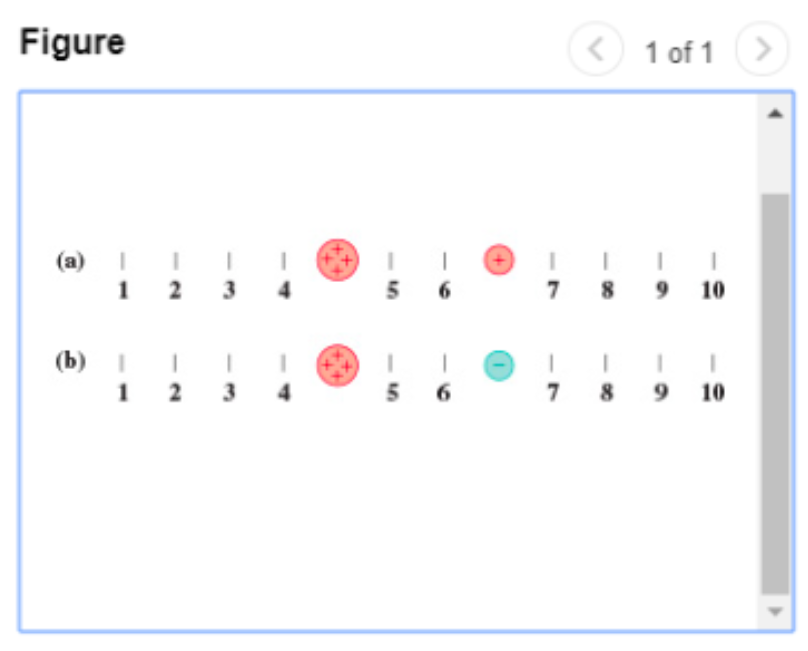# Problem: Two pairs of charges are shown in (Figure 1)Part A. At which point or points is the electric field zero in (Figure 1)(a)?Part B. At which point or points is the electric field zero in (Figure 2)(b)?

###### FREE Expert Solution

In this problem, were are dealing with sum of electric field vectors. Electric field points radially outward from a positive charge and radially inward to a negative charge.

96% (344 ratings)###### Problem Details

Two pairs of charges are shown in (Figure 1)Part A. At which point or points is the electric field zero in (Figure 1)(a)?

Part B. At which point or points is the electric field zero in (Figure 2)(b)?